Matlab is a programming platform used to analyse data, create algorithms and also create models. Tables in Matlab are used to make storing and reading of data more efficient and understandable. They consist of rows and column-oriented variables.

All the variables in the table can be of different sizes and data types, ensuring that all the variables have an equal number of rows.

The table is a data type used for tabular data. It is one of the most effective and efficient ways to summarise any given information in columns, which also helps find specific information easily.

# Tables in MATLAB

Tables store the column-oriented data in a variable. Often table, table array, and matrix are confused in Matlab. They are similar with just a slight difference in their characteristics.

## Table and Array Table

As mentioned above, they consist of rows and column-oriented variables and store column-oriented data like columns from any text file or spreadsheet directly. The table variables can be of different data types and sizes, though a specific column must contain all the same data type variables. The only constraint in this is that the number of rows needs to be the same throughout. Table and Table array are the same thing.

## Martix and Array

Matlab stands for Matrix Laboratory, and hence it is primarily designed to perform matrix operations. A matrix or array should have the data type of all the variables to be the same even in different columns. This means that the entire matrix or array will be of only one data type. Matrices aren’t as memory efficient as tables.

Also Read: Bash functions explained

# How to create a table in MATLAB?

We already know that working with tables improves efficiency and the capability to understand the data. They are vital for better readability of tables and increased efficiency in understanding the data.

The methods for creating tables discussed below use the following functions:

With these simple functions, the most complex array, cell or structure can be converted into a table, with and without the variable names depending on the syntax. Listed below are the different ways of creating a table in Matlab.

## Using keyword ‘table’

The keyword ‘table’ creates a table array with named variables that can contain different types. The syntax for the table is explained in the following example.

Here, all the variables with their specific data are initialised. A table named T is then declared and assigned the values of all the variables initialised previously. When this code is run, the output obtained is a 6×4 table.

The output table has a mix of data types standing true to its property.

## Using Function array2table

The function array2table is used to convert a homogeneous array to a table. There are two possible conversions using this method.

Without Variable Names

A matrix A has been declared in the syntax below, and a table named T. Table T is assigned to the function array2table to convert matrix A into a table.

The output for this code is observed as a 4×4 table without any specific variable names given. Since the code did not specify any variable names, the table takes the variable names to be A1, A2, A3, and A4.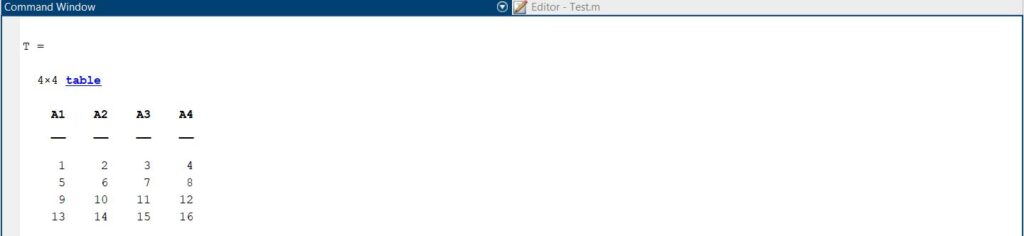Output for array to table conversion without variable name

To assign variable names to this, follow the method below.

With Variable Names

The syntax for printing the table from an array with variable names is very similar to the syntax without variable names. The only key difference is in using the function array2table assigned to Table T.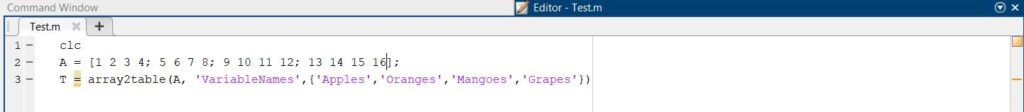Syntax for array to table conversion with variable names

Here, since the variable names were given, the output does not have the names A1, A2, A3, and A4 like it did previously. Instead, it has the variable names as that of fruits.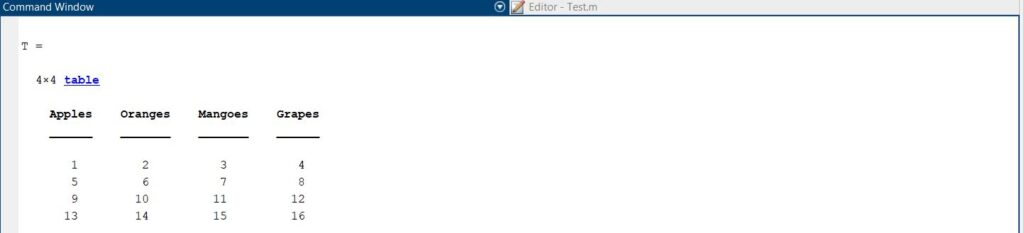Output for array to table conversion with variable name

Also, note that in this code, one of the numbers is in the float data type (8.7), but this has caused no error since the upper-class data type is still numeric.

## Using Function cell2table

The function cell2table is used to convert a cell array to a table. Just like the array2table function, this can also be done in two ways.

Without Variable Names

The following syntax for converting a cell A predefined with values to a table T using the function cell2table.

Similar to the functioning of array2table, the table can get misleading without variable names, hence defying the table’s purpose completely.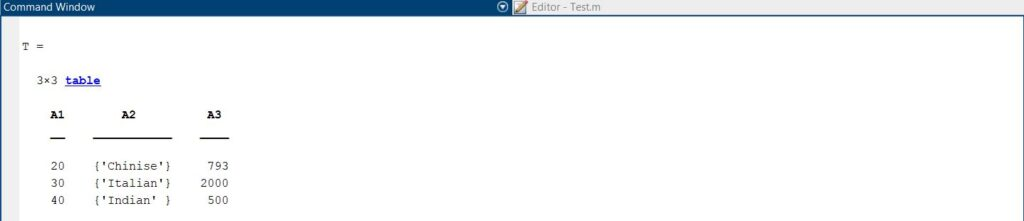Output for cell to table conversion without variable name

To assign variable names, the method below can be followed.

With Variable Names

The following syntax is used to convert cell A into a table T with the variable names for each column.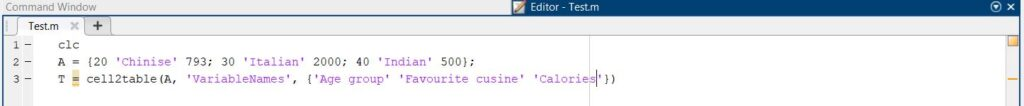Syntax for cell to table conversion with Variable Names

It can be clearly observed that the table’s readability is maximised.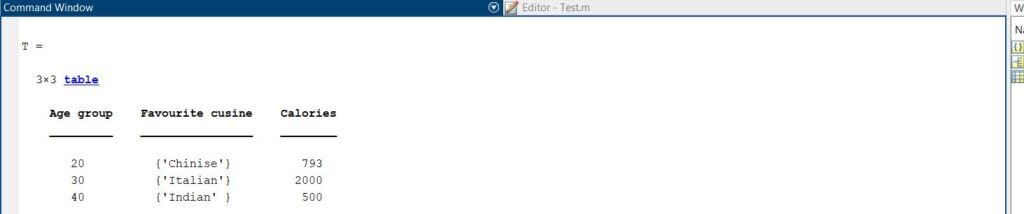Output for cell to table conversion with variable name

## Using Function struct2table

The function struct2table is used to convert a structure array to a table. There can be two types of structure arrays while converting it to a table. The syntax for the function struct2table for both types of structure arrays is below.

Scalar Structure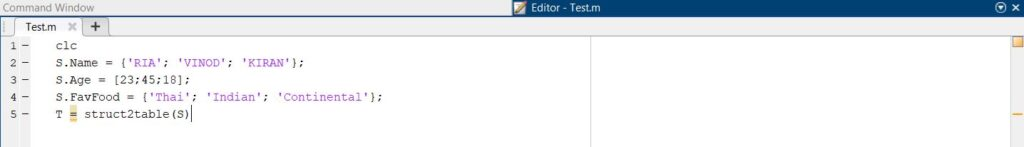Syntax for structure to table conversion for Scalar Structure

The above code shows the initialisation of the Scalar Structure, ‘S’. T is the table’s declaration, which uses the function struct2table is converting the structure to a tabular form, as shown below.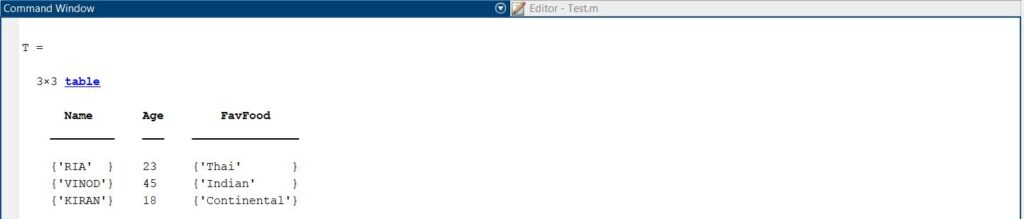Output for structure to table conversion for Scalar Structure

Due to the initialisation of the variables, while giving the data, the table is not unnamed as A1, A2 and A3 as seen in our previous case of array2table and cell2table.

Non-Scalar Structure

The following code represents the syntax for the conversion of a non-scalar structure to a table using the struct2table function.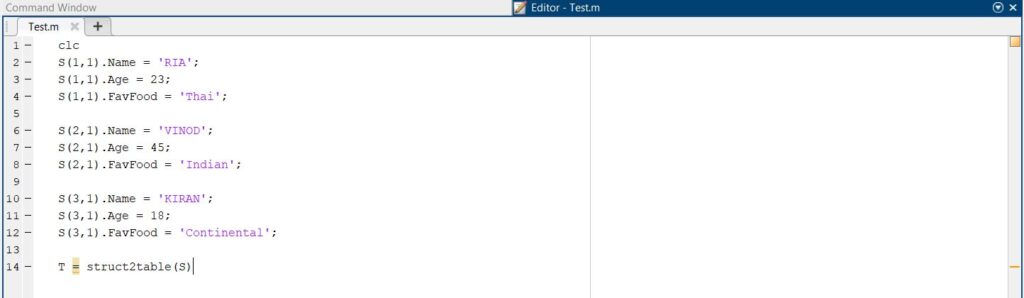Syntax for structure to table conversion for Non-Scalar Structure

Following is the output table for a non-scalar structure. The output obtained in both cases is the same.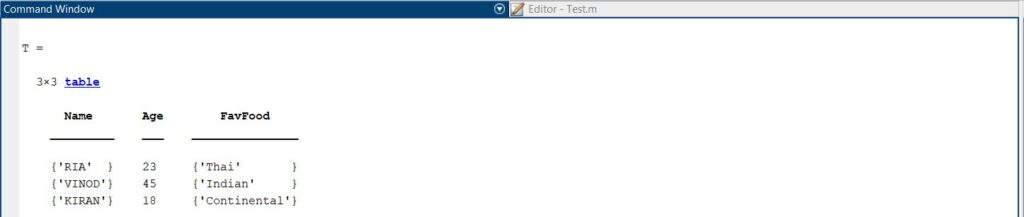Output for structure to table conversion for Non-Scalar Structure

Also Read: How to concatenate strings in Python?

## Read more from Candid.Technology

Copyright © 2018-2022 Candid.Technology | Candid Today Press Pvt. Ltd.​